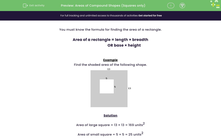# Find the Area of Part of a Square

In this worksheet, students will find areas of compound shapes made from squares.Key stage:  KS 3

Curriculum topic:   Geometry and Measures

Curriculum subtopic:   Solve Problems Involving Perimeters and Areas of 2D Shapes

Popular topics:   Area worksheets, Geometry worksheets

Difficulty level:#### Worksheet Overview

You need to know the formula for finding the area of a rectangle:

Area of a rectangle = length × breadth

OR base × height

Example

Find the shaded area of the following shape.Solution

Area of large square = 13 × 13 = 169 units2

Area of small square = 5 × 5 = 25 units2

Shaded area = 169 − 25 = 144 units2

Did that make sense?On to the questions!

### What is EdPlace?

We're your National Curriculum aligned online education content provider helping each child succeed in English, maths and science from year 1 to GCSE. With an EdPlace account you’ll be able to track and measure progress, helping each child achieve their best. We build confidence and attainment by personalising each child’s learning at a level that suits them.

Get started